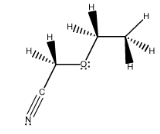# Problem: Convert the following hashed-wedged line formula into its condensed formula.

###### FREE Expert Solution

We are asked to convert the following hashed-wedged line formula into its condensed formula.

The condensed structure shows us the connectivity of the molecule.

The use of parentheses is important:

• Parentheses with no subscripts: Branch on the chain.
• Parenthesis with subscripts: Multiple branches on a chain.
• CHwithin parentheses + subscripts: Repeating CHunits within a chain.###### Problem Details

Convert the following hashed-wedged line formula into its condensed formula.